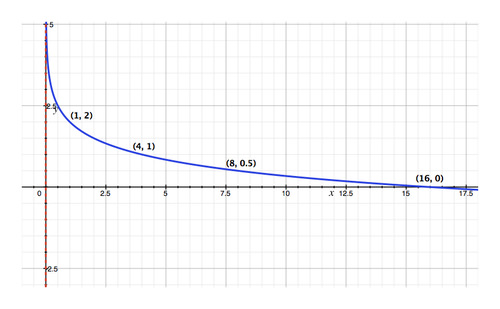Skip to main content

# Graphing Logarithmic Functions

Your math homework assignment is to find out which quadrants the graph of the function f(x)=4ln(x+3) falls in. On the way home, your best friend tells you, "This is the easiest homework assignment ever! All logarithmic functions fall in Quadrants I and IV." You're not so sure, so you go home and graph the function as instructed. Your graph falls in Quadrant I as your friend thought, but instead of Quadrant IV, it also falls in Quadrants II and III. Which one of you is correct?

# Graphing Logarithmic Functions

Now that we are more comfortable with using these functions as inverses, let’s use this idea to graph a logarithmic function. Recall that functions are inverses of each other when they are mirror images over the line y=x. Therefore, if we reflect y=bx over y=x, then we will get the graph of y=logbx.

Recall that an exponential function has a horizontal asymptote. Because the logarithm is its inverse, it will have a vertical asymptote. The general form of a logarithmic function is f(x)=a logb(x−h)+k and the vertical asymptote is x=h. The domain is x>h and the range is all real numbers. Lastly, if b>1, the graph moves up to the right. If 0<b<1, the graph moves down to the right.

Let's graph y=log3(x−4) and state the domain and range.To graph a logarithmic function without a calculator, start by drawing the vertical asymptote, at x=4. We know the graph is going to have the general shape of the first function above. Plot a few points, such as (5, 0), (7, 1), and (13, 2) and connect.

The domain is x>4 and the range is all real numbers.

Now, let's determine if (16, 1) is on y=log(x−6).

Plug in the point to the equation to see if it holds true.

$$\ \begin{array}{l} 1=\log (16-6) \\ 1=\log 10 \\ 1=1 \end{array}$$

Yes, this is true, so (16, 1) is on the graph.

Finally, let's graph f(x)=2ln(x+1).

To graph a natural log, we can use a graphing calculator. Press Y= and enter in the function, Y=2ln(x+1), GRAPH.# Examples

Example 1

Earlier, you were asked to determine if your friend was correct.

Solution

The vertical asymptote of the function f(x)=4ln(x+3) is x=−3. Since x will approach −3 but never quite reach it, x can assume some negative values. Hence, the function will fall in Quadrants II and III. Therefore, you are correct and your friend is wrong.

Example 2

Graph $$\ y=\log _{\frac{1}{4}} x+2$$ in an appropriate window.

Solution

First, there is a vertical asymptote at x=0. Now, determine a few easy points, points where the log is easy to find; such as (1, 2), (4, 1), (8, 0.5), and (16, 0).To graph a logarithmic function using a TI-83/84, enter the function into Y= and use the Change of Base Formula: $$\ \log _{a} x=\frac{\log _{b} x}{\log _{b} a}$$. The keystrokes would be: $$\ Y=\frac{\log (x)}{\log \left(\frac{1}{4}\right)}+2$$, GRAPH

To see a table of values, press 2nd→ GRAPH.

Example 3

Graph y=−logx using a graphing calculator. Find the domain and range.

Solution

The keystrokes are Y=−log(x), GRAPH.The domain is x>0 and the range is all real numbers.

Example 4

Is (-2, 1) on the graph of $$\ f(x)=\log _{\frac{1}{2}}(x+4)$$?

Solution

Plug (-2, 1) into $$\ f(x)=\log _{\frac{1}{2}}(x+4)$$ to see if the equation holds true.

$$\ \begin{array}{l} 1=\log _{\frac{1}{2}}(-2+4) \\ 1=\log _{\frac{1}{2}} 2 \\ 1 \neq-1 \end{array}$$

Therefore, (-2, 1) is not on the graph. However, (-2, -1) is.

# Review

Graph the following logarithmic functions without using a calculator. State the equation of the asymptote, the domain and the range of each function.

1. $$\ y=\log _{5} x$$
2. $$\ y=\log _{2}(x+1)$$
3. $$\ y=\log (x)-4$$
4. $$\ y=\log _{\frac{1}{3}}(x-1)+3$$
5. $$\ y=-\log _{\frac{1}{2}}(x+3)-5$$
6. $$\ y=\log _4(2-x)+2$$

Graph the following logarithmic functions using your graphing calculator.

1. $$\ y=\ln(x+6)-1$$
2. $$\ y=-\ln(x-1)+2$$
3. $$\ y=\log(1-x)+3$$
4. $$\ y=\log(x+2)-4$$
5. How would you graph $$\ y=\log_4x$$ on the graphing calculator? Graph the function.
6. Graph $$\ y=\log_{\frac{3}{4}}x$$ on the graphing calculator.
7. Is (3, 8) on the graph of $$\ y=\log_3(2x-3)+7$$?
8. Is (9, -2) on the graph of $$\ y=\log_{\frac{1}{4}}(x-5)$$?
9. Is (4, 5) on the graph of $$\ y=5\log_2(8-x)$$?

# Answers for Review Problems

To see the Review answers, open this PDF file and look for section 8.7.

# Vocabulary

Term Definition
Asymptotes An asymptote is a line on the graph of a function representing a value toward which the function may approach, but does not reach (with certain exceptions).
operation Operations are actions performed on variables, constants, or expressions. Common operations are addition, subtraction, multiplication, and division.

# Image Attributions

1. [Figure 1]
Credit: CK-12
Source: CK-12
2. [Figure 2]
Credit: CK-12
Source: CK-12
3. [Figure 3]
Credit: CK-12 Foundation
• Was this article helpful?